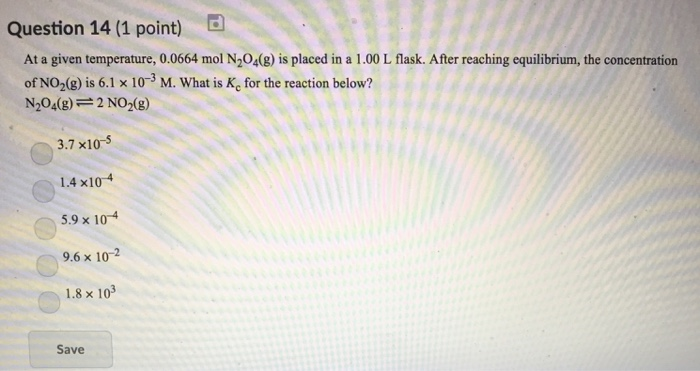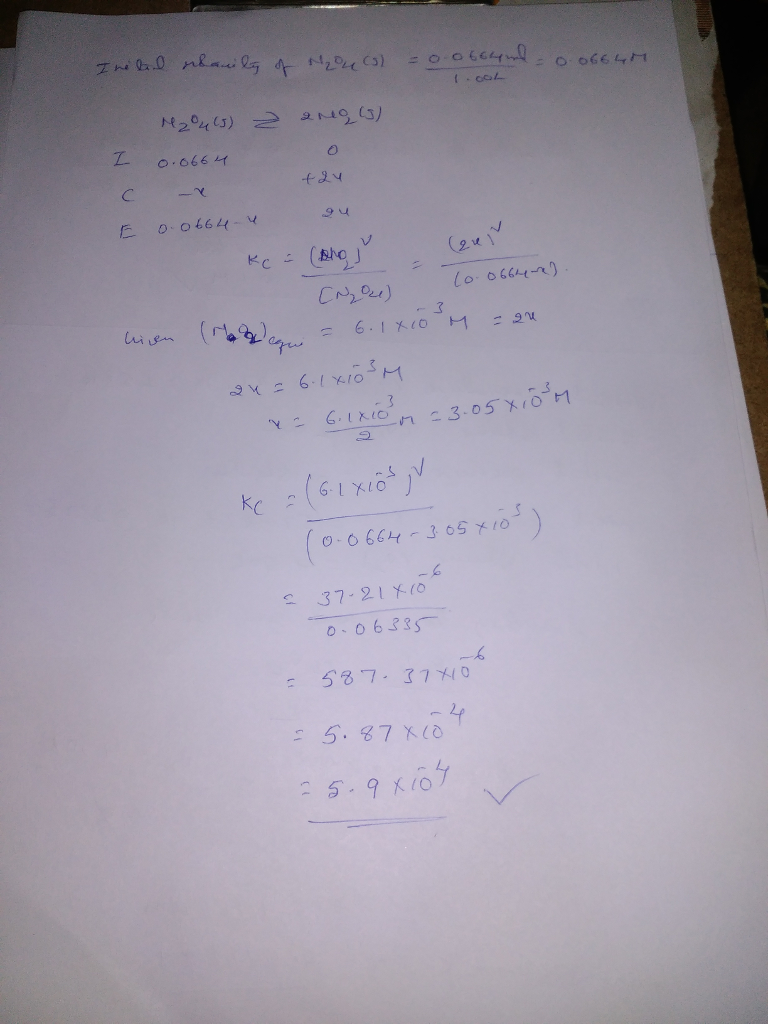# Question & Answer: At a given temperature, 0.0664 mol N_2O_4(g) is placed in a 1.00 L flask. After reaching…..At a given temperature, 0.0664 mol N_2O_4(g) is placed in a 1.00 L flask. After reaching equilibrium, the concentration of NO_2(g) is 6.1 times 10^-3 M. What is K_c for the reaction below? N_2O_4(g) rlhar 2 NO_2(g) 3.7 times10^-5 1.4 times10^-4 5.9 times 10^-4 9.6 times 10^-2 1.8 times 10^3 Save